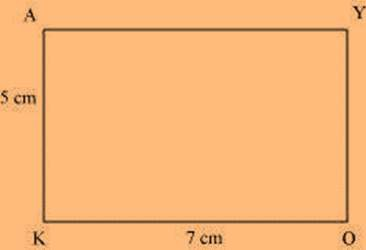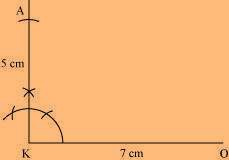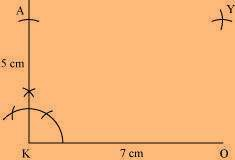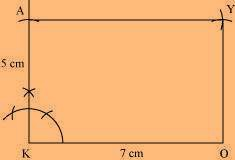# Construct the following quadrilateral: Rectangle OKAY OK = 7 cm KA = 5 cm

SolutionWe can draw the quadrilateral OKAY using the steps given below

(1) Draw a line segment OK of 7 cm and an angle of 90º at point K. As vertex A is 5 cm away from vertex K, cut a line segment KA of 5 cm from this ray.(2) Vertex Y is 5 cm and 7 cm away from vertex O and A respectively. Considering radii as 5 cm and 7 cm, draw arcs from points O and A respectively. These will be intersecting each other at point Y.(3) Join Y to A and O.(0)(0)